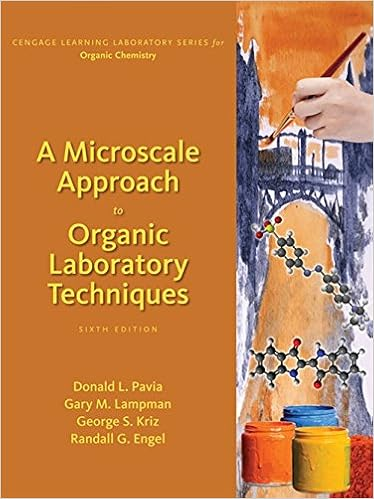OxalateTitration - CHEM 121L General Chemistry Laboratory...

• 10

This preview shows page 1 - 3 out of 10 pages.

We have textbook solutions for you!
The document you are viewing contains questions related to this textbook.The document you are viewing contains questions related to this textbook.
Chapter 3 / Exercise 2
A Microscale Approach to Organic Laboratory Techniques
Kriz/PaviaExpert Verified
CHEM 121L General Chemistry Laboratory Revision 3.0 The Determination of Oxalate Ion in Ferric Oxalate Trihydrate using Titrimetry Learn how to perform a quantitative chemical analysis. Learn how to perform a titration. Learn about Oxidation-Reduction reactions. In this laboratory exercise, we will determine the Percentage Oxalate Ion (C 2 O 4 2- ) in the Potassium Ferric Oxalate Trihydrate (K 3 [Fe(C 2 O 4 ) 3 ] 3H 2 O) sample we synthesized previously. This will be accomplished using a technique called Titration; a type of Volumetric Analysis. This type of analysis involves adding a Potassium Permanganate (KMnO 4 ) solution, whose concentration is previously determined, to a solution of the Oxalate until the reaction between these species is complete. By knowing the reaction stoichiometry, the volume Permanganate Ion (MnO 4 - ) solution required for complete reaction, and the concentration of the solution used, we can quantitatively determine the mass percentage of Oxalate Ion present in our sample. This can then be compared with the expected mass percentage Oxalate based on our compound's chemical formula and therefore allow us to confirm or discount the solid we have generated is indeed the trioxalate of Iron. A volumetric analysis involves measuring the volume of a solution of known concentration, the Titrant, Permanganate Ion in the present case, which is needed to completely react with an Analyte, the Oxalate Ion of our Ferric Oxalate sample. 5 C 2 O 4 2- (aq) + 2 MnO 4 - (aq) + 16 H + (aq) 10 CO 2 (g) + 2 Mn 2+ (aq) + 8 H 2 O (Eq. 1) The titration reaction is complete when a stoichiometrically equivalent amount of titrant has been added to the analyte and all the analyte has been consumed; a point in the titration called the Equivalence Point. To be successful things must be arranged so that some distinct visual change occurs in the analyte solution at the Equivalence Point. The point at which this visual change occurs is called the Endpoint of the titration. (But, shouldn't the Endpoint be the same as the Equivalence Point? Yes, and it is the job of the analyst to pick a visual indicator that will ensure this is so.) The trick in any titration is to stop adding titrant at the exact point the Endpoint is reached because it is only at this point that a stoichiometrically correct amount of titrant has been added to the analyte.
We have textbook solutions for you!
The document you are viewing contains questions related to this textbook.The document you are viewing contains questions related to this textbook.
Chapter 3 / Exercise 2
A Microscale Approach to Organic Laboratory Techniques
Kriz/PaviaExpert Verified
P a g e | 2 A Buret is the volume measuring device we will use to deliver the titrant solution into the analyte Solution. A Graduated 50 mL Burette () This device is constructed such that it can be read with a high degree of accuracy. Accepted tolerances for burets are listed in the Appendix below.
•••